# 什么是关系代数

1、概念

2、分类

$\cup$
$\cap$
$-$
×$\times$ 笛卡儿积

σ$\sigma$ 选择
Π$\Pi$ 投影
$连接$ 连接
÷$\div$

## 集合运算符

1、并 : R∪S = { t| tR$t \in R$tS$t \in S$ }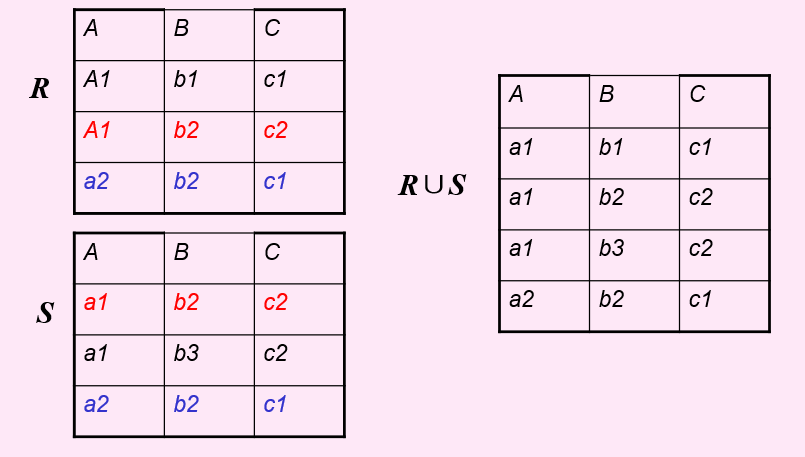2、差 : R-S = { t| tR$t \in R$tS$t \notin S$ }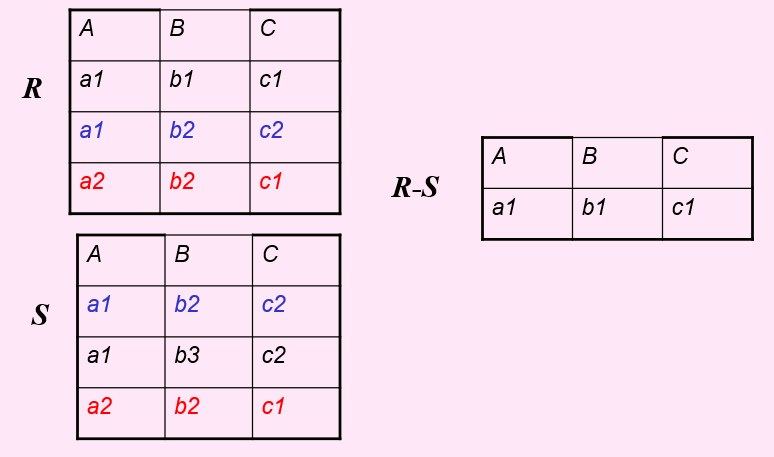3、交 : R∩S = { t| tR$t \in R$tS$t \notin S$ }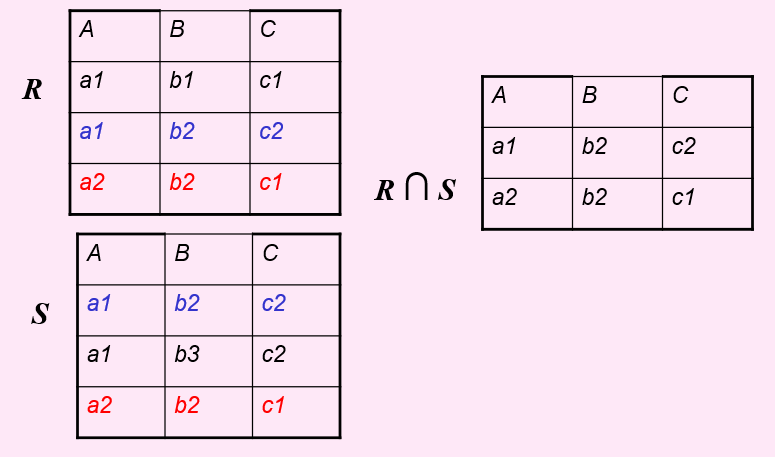4、笛卡儿积 :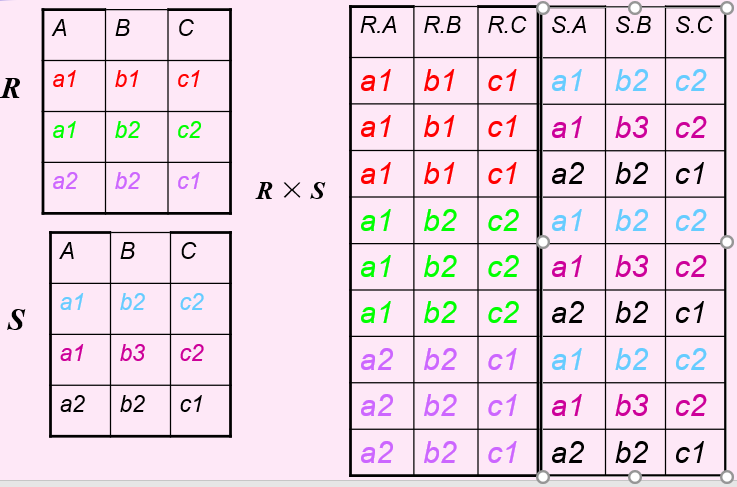## 专门的关系运算

R

X Z
x1 z2
x1 z3
x1 z1
x2 z2
x2 z3

1、选择 (从行的角度进行的运算）

σF(R) = {t|tR$t \in R$∧F(t)= ‘真’}
F是一个选择条件，即从R中选出满足F为真的行。

2、投影 （从列的角度进行的运算）

Π$\Pi$A(R) = { t[A] | tR$t \in R$ }

3、连接
（连接的符号太难表示了呜呜呜。。。）
(1) 等值连接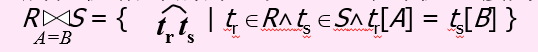(2) 自然连接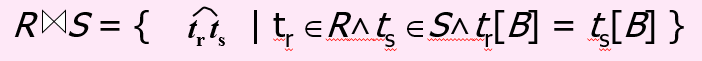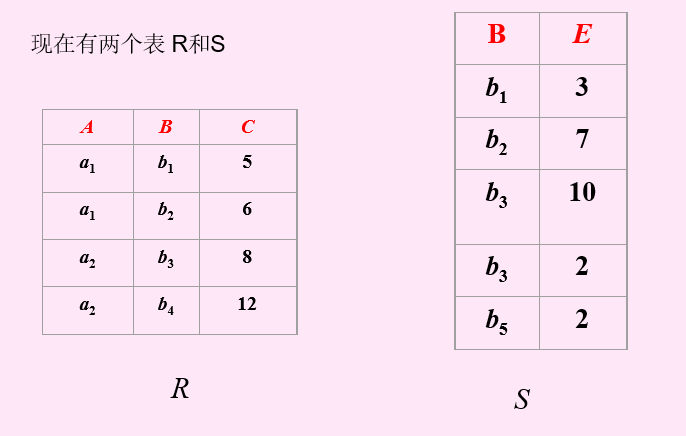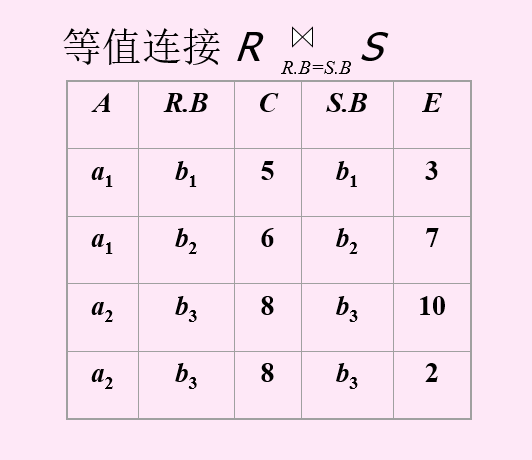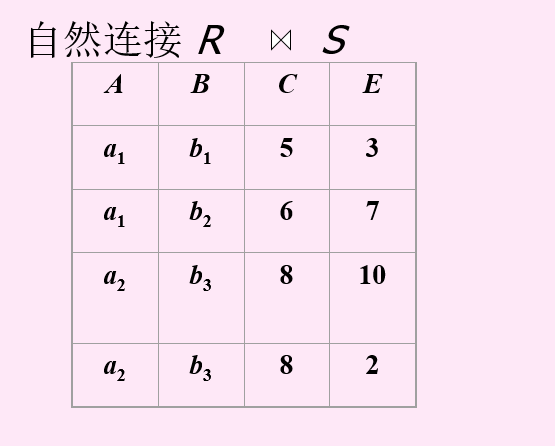R×S$R \times S$ 中挑选符合C＜E的元组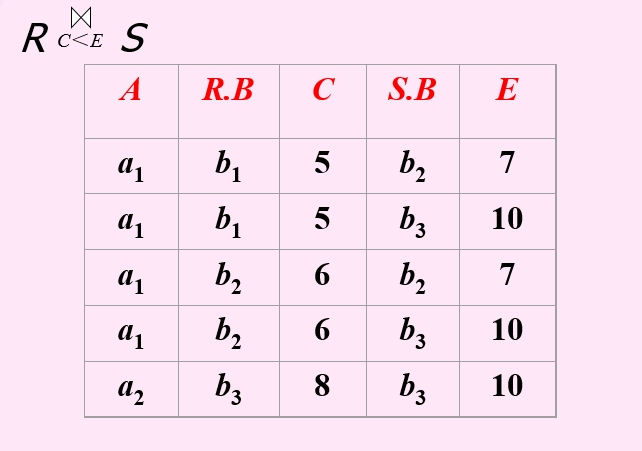4、除运算

1、查询在Student表中CS系的学生。
2、查询Student表中学号属性。
3、查询Student表中CS系的学生的学号。
4、在Student表中查询SC表中成绩大于等于90的学生的姓名。
（《数据库系统概论》P52表）。